# Python Program to Add Two Numbers

In this tutorial you will learn about the Python Program to Add Two Numbers and its application with practical example.

In this tutorial, we will learn to create a Python Program to add two Numbers using python Programming.

## Prerequisites

Before starting with this tutorial we assume that you are best aware of the following Python programming topics:

• Python Operator.
• Basic Input and Output
• Basic Python  programming.
• Python Data type.

## What are Operators.?

They are special symbols that carry out arithmetic and logical  calculation. Values that the Operator handle on is called the operand.

## Python Program to Add Two Numbers.

In our program , we will add two values.First we would declared and initialized the required variables. Next, we would prompt user to input values. Later we will add them.

## Output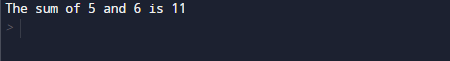Here, ” + “is a Operator that do addition. 5 and 6 are the Operands and “11” is the output of our calculations.

Now the program for calculates the sum of two numbers entered by the user.

## Output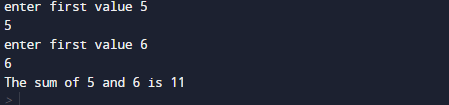In our program, we have first declared and initialized a set variables required in the program.

• a =taking a value from user.
• b=taking second value from user .

First of all  we declare variable and take two values from user and assign in variables ‘a’ and ‘b’ respectively.

In our program, we will ask user to enter two values and displays the sum of two numbers entered by user.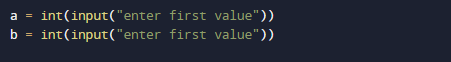Here we use the built-in method of python “input()” to take the input from user. Since,the value returned bye the built-in method “input()” is string,So we need to convert the string  value into number using “int()” function. Then,afterward we add these numbers.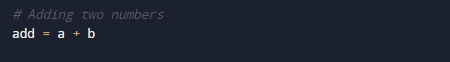So in our program above we calculates the sum of two numbers entered by the user.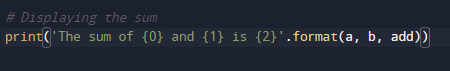In this tutorial we have learn about the Python Program to Add Two Numbers and its application with practical example. I hope you will like this tutorial.